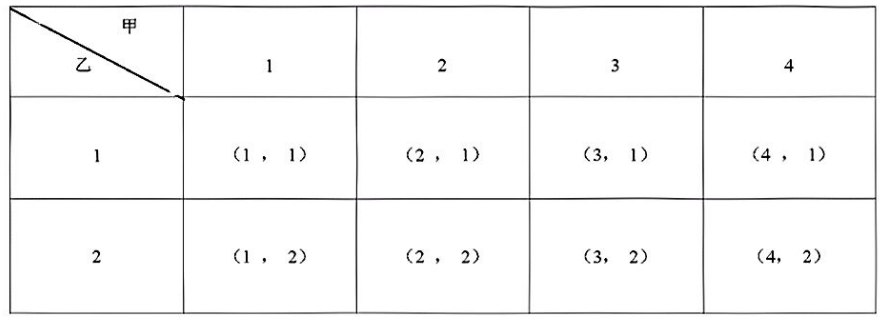（1）用列表法或画树状图法中的一种方法，求（a，b）所有可能出现的结果总数;
（2）你认为这个游戏公平剪?如果公平，请说明理由;如果不公平，哪一首乐曲更可

【答案】 （1）列表分析如下(2) 我认为这个游戏公平的, 理由如下:

$$\therefore P(A)=\frac{4}{8}=\frac{1}{2} \quad, \quad P(B)=\frac{4}{8}=\frac{1}{2} \quad \text { 即 } P(A)=P(B)=\frac{1}{2}$$
$\therefore$ 这个游戏公平.